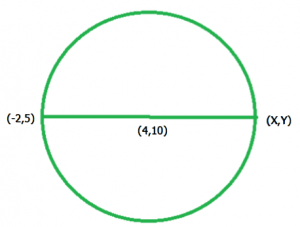GeeksforGeeks App
Open AppBrowser
Continue

# Find the other-end coordinates of diameter in a circle

Given Centre coordinate (c1, c2) and one coordinate (x1, y1) of the diameter of a circle, find the other end coordinate point (x2, y2) of diameter.Examples:

Input  : x1 = –1, y1 = 2, and c1 = 3, c2 = –6
Output : x2 = 7, y2 = -14

Input  : x1 = 6.4, y1 = 3 and c1 = –10.7, c2 = 4
Output : x2 = -27.8, y2 = 5

The Midpoint Formula:
The midpoint of two ends coordinates points, (x1, y2) and (x2, y2) is the point M can be found by using:We have need of a (x2, y2) coordinates, so we apply the midpoint the formula

          c1 = ((x1+x2)/2),  c2 = ((y1+y2)/2)
2*c1 = (x1+x2),  2*c2 = (y1+y2)
x2 = (2*c1 - x1),  y2 = (2*c2 - y1)

## C++

 // CPP program to find the// other-end point of diameter#include using namespace std; // function to find the// other-end point of diametervoid endPointOfDiameterofCircle(int x1,                    int y1, int c1, int c2){    // find end point for x coordinates    cout << "x2 = "            << (float)(2 * c1 - x1)<< "  ";         // find end point for y coordinates    cout << "y2 = " << (float)(2 * c2 - y1);     }// Driven Programint main(){    int x1 = -4, y1 = -1;    int c1 = 3, c2 = 5;         endPointOfDiameterofCircle(x1, y1, c1, c2);         return 0;}

## Java

 // Java program to find the other-end point of// diameterimport java.io.*; class GFG {         // function to find the other-end point of    // diameter    static void endPointOfDiameterofCircle(int x1,                        int y1, int c1, int c2)    {                 // find end point for x coordinates        System.out.print( "x2 = "                            + (2 * c1 - x1) + " ");                 // find end point for y coordinates        System.out.print("y2 = " + (2 * c2 - y1));    }         // Driven Program    public static void main (String[] args)    {        int x1 = -4, y1 = -1;        int c1 = 3, c2 = 5;                 endPointOfDiameterofCircle(x1, y1, c1, c2);    }} // This code is contributed by anuj_67.

## Python3

 # Python3 program to find the# other-end point of diameter # function to find the# other-end point of diameterdef endPointOfDiameterofCircle(x1, y1, c1, c2):     # find end point for x coordinates    print("x2 =", (2 * c1 - x1), end=" ")         # find end point for y coordinates    print("y2 =" , (2 * c2 - y1))     # Driven Programx1 = -4y1 = -1c1 = 3c2 = 5     endPointOfDiameterofCircle(x1, y1, c1, c2)     # This code is contributed by Smitha.

## C#

 // C# program to find the other -// end point of diameterusing System;class GFG {         // function to find the other - end    // point of  diameter    static void endPointOfDiameterofCircle(int x1,                                           int y1,                                           int c1,                                           int c2)    {        // find end point for x coordinates        Console.Write("x2 = "+ (2 * c1 - x1) + " ");                 // find end point for y coordinates        Console.Write("y2 = " + (2 * c2 - y1));    }         // Driver Code    public static void Main ()    {        int x1 = -4, y1 = -1;        int c1 = 3, c2 = 5;                 endPointOfDiameterofCircle(x1, y1, c1, c2);    }} // This code is contributed by anuj_67.

## PHP

 

## Javascript

 

Output

x2 = 10 y2 = 11

Time complexity: O(1)

Auxiliary Space: O(1)
Similarly if we given a centre (c1, c2) and other end coordinate (x2, y2) of a diameter and we finding a (x1, y1) coordinates

 Proof for (x1, y1) :
c1 = ((x1+x2)/2),  c2 = ((y1+y2)/2)
2*c1 = (x1+x2),  2*c2 = (y1+y2)
x1 = (2*c1 - x2),  y1 = (2*c2 - y2)

So The other end coordinates (x1, y1) of a diameter is

         x1 = (2*c1 - x2),  y1 = (2*c2 - y2)

My Personal Notes arrow_drop_up